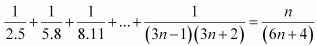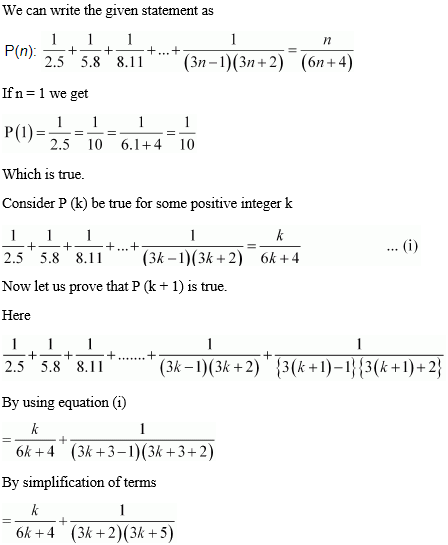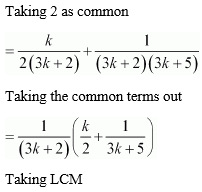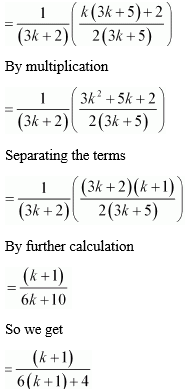• +91 9971497814
• info@interviewmaterial.com

# Chapter 4 Principle of Mathematical Induction Ex-4.1 Interview Questions Answers

### Related Subjects

Question 1 : Prove the following by using the principle of mathematical induction for all n ∈ N: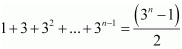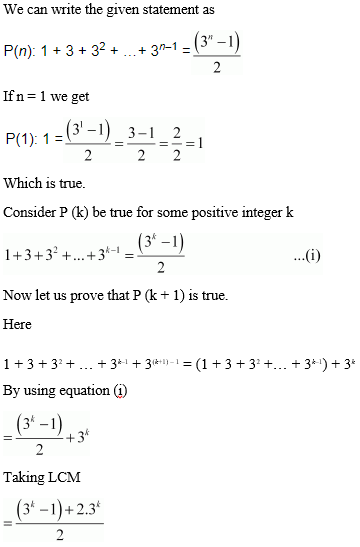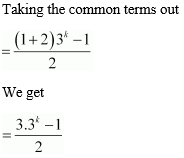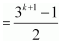P (k + 1) is true whenever P (k) is true.
Therefore, by the principle of mathematical induction, statement P (n) is true for all natural numbers i.e. n.

Question 2 : Prove the following by using the principle of mathematical induction for all n ∈ N: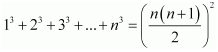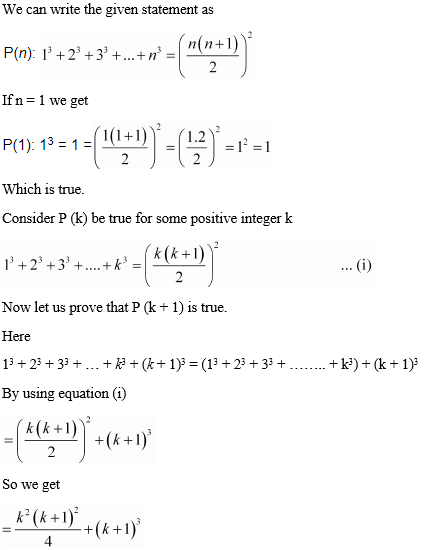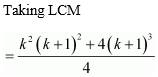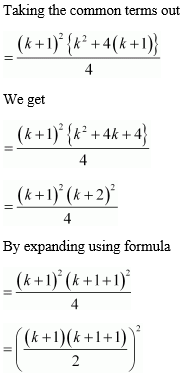P (k + 1) is true whenever P (k) is true.
Therefore, by the principle of mathematical induction, statement P (n) is true for all natural numbers i.e. n.

Question 3 : Prove the following by using the principle of mathematical induction for all n ∈ N: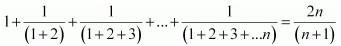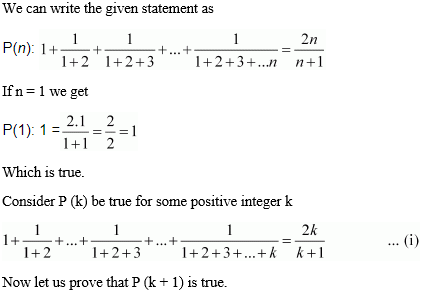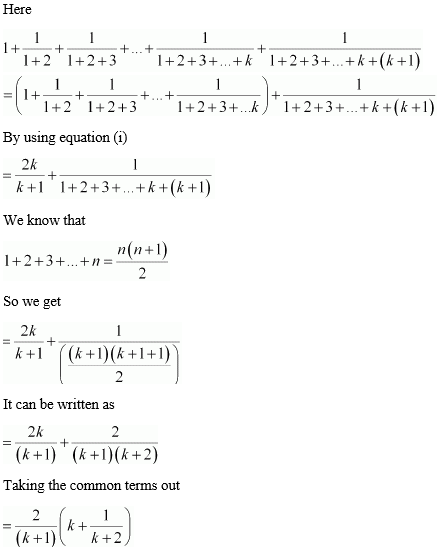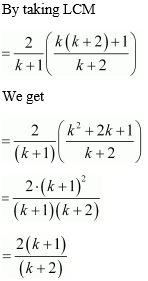P (k + 1) is true whenever P (k) is true.
Therefore, by the principle of mathematical induction, statement P (n) is true for all natural numbers i.e. n.

Question 4 : Prove the following by using the principle of mathematical induction for all n ∈ N: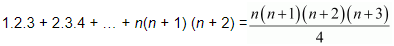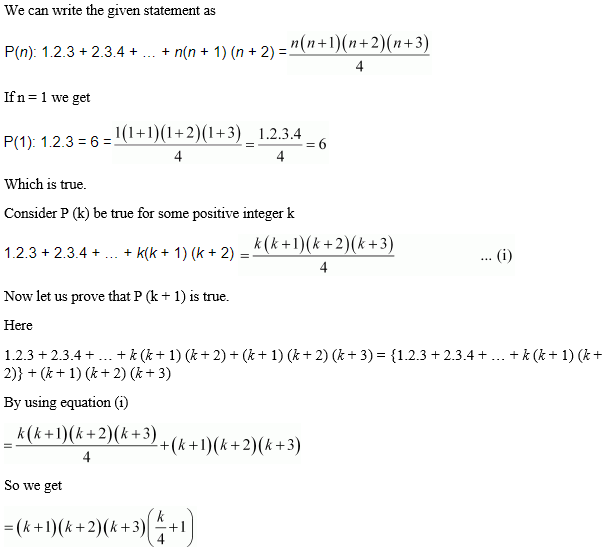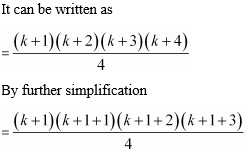P (k + 1) is truewhenever P (k) is true.

Therefore, by theprinciple of mathematical induction, statement P (n) is true for all naturalnumbers i.e. n.

Question 5 :
Prove the following by using the principle of mathematical induction for all n ∈ N: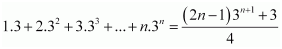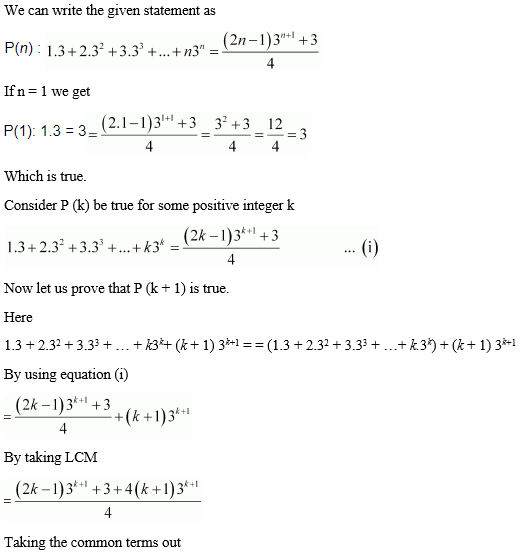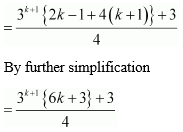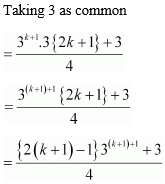P (k + 1) is true whenever P (k) is true.
Therefore, by the principle of mathematical induction, statement P (n) is true for all natural numbers i.e. n.

Question 6 :
Prove the following by using the principle of mathematical induction for all n ∈ N: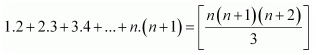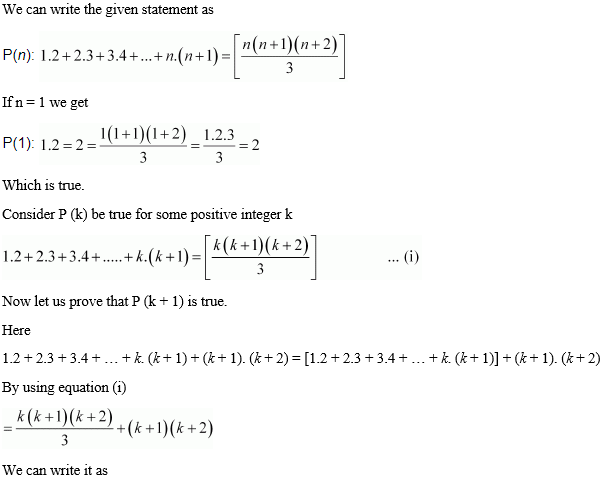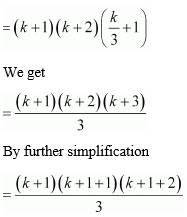P (k + 1) is true whenever P (k) is true.
Therefore, by the principle of mathematical induction, statement P (n) is true for all natural numbers i.e. n.

Question 7 :
Prove the following by using the principle of mathematical induction for all n ∈ N: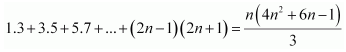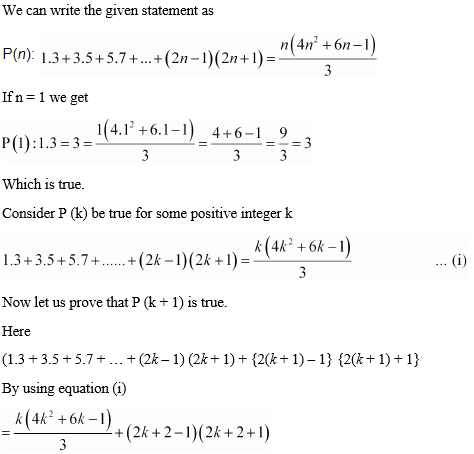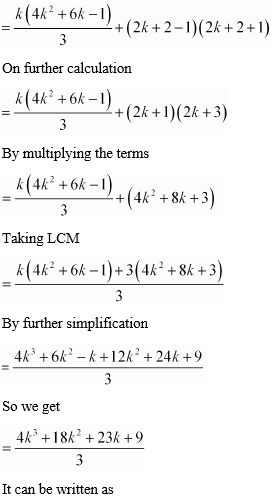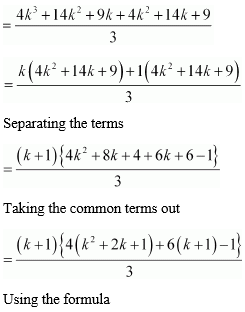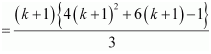P (k + 1) is true whenever P (k) is true.
Therefore, by the principle of mathematical induction, statement P (n) is true for all natural numbers i.e. n.

Question 8 :
Prove the following by using the principle of mathematical induction for all n ∈ N:

1.2 + 2.22 + 3.22 + … + n.2n =(n – 1) 2n+1 + 2

We can write the givenstatement as

P (n): 1.2 +2.22 + 3.22 + … + n.2n =(n – 1) 2n+1 + 2

If n = 1 we get

P (1): 1.2 = 2 = (1 –1) 21+1 + 2 = 0 + 2 = 2

Which is true.

Consider P (k) be truefor some positive integer k

1.2 + 2.22 +3.22 + … + k.2k = (k –1) 2k + 1 + 2 … (i)

Now let us prove thatP (k + 1) is true.

Here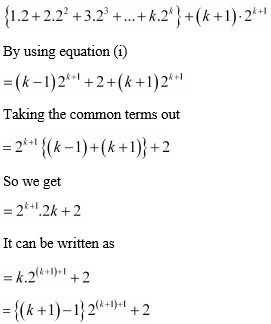P (k + 1) is truewhenever P (k) is true.

Therefore, by theprinciple of mathematical induction, statement P (n) is true for all naturalnumbers i.e. n.

Question 9 :
Prove the following by using the principle of mathematical induction for all n ∈ N: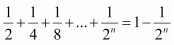We can write the givenstatement as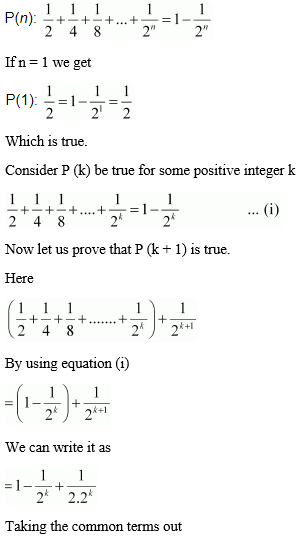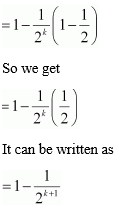P (k + 1) is truewhenever P (k) is true.

Therefore, by theprinciple of mathematical induction, statement P (n) is true for all naturalnumbers i.e. n.

Question 10 :
Prove the following by using the principle of mathematical induction for all n ∈ N: